# Arithmetic derivative

This article defines an arithmetic function or number-theoretic function: a function from the natural numbers to a ring (usually, the ring of integers, rational numbers, real numbers, or complex numbers).
View a complete list of arithmetic functions

## Definition

The arithmetic derivative or number derivative is an arithmetic function, specifically a function from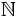to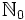denoted by thesuperscript, defined in a number of equivalent ways.

Definition type Definition details
using Leibniz rule and specification on primes It is defined by the following three conditions: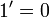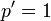for any prime numberLeibniz rule: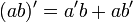for any (possibly equal, possibly distinct) natural numbersdirect definition in terms of prime factorization Consider a natural numberwith prime factorizationwhere theare all distinct primes and the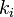are all positive integers (possibly repeated). Then the arithmetic derivativeis given by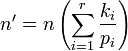## Higher derivatives

Note that for any, the arithmetic derivative ofis nonzero, so the arithmetic derivative operation can be iterated for. We can thus consider iterations of the arithmetic derivative operation, which are denoted by using multiple primes. Note that higher derivatives make sense only as long as we do not hit zero.

The second derivative of, for instance, is denoted.

## Relation with conjectures

Conjecture What the conjecture, if true, would imply about the arithmetic derivative Explanation Is the converse implication true?
Goldbach's conjecture: every even integer greater than 2 is expressible as a sum of two primes. For every even integerwith, there exists an integersuch that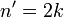. If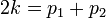, then we can take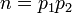. Note that this construction works regardless of whetherare equal or distinct. Not obviously so
twin primes conjecture: there exist infinitely many primesfor which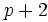is prime. There exist infinitely many integerssuch that. For any primesuch thatis prime,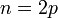satisfies. Not obviously so